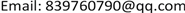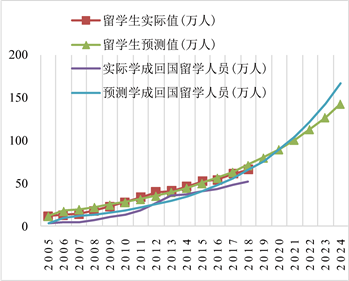﻿ 基于灰色预测及改进模型的留学人员预测 Forecast of Overseas Students Based on Grey Prediction and Improvement Model

Statistics and Application
Vol. 08  No. 04 ( 2019 ), Article ID: 31882 , 8 pages
10.12677/SA.2019.84079

Forecast of Overseas Students Based on Grey Prediction and Improvement Model

Tianhao Jin, Shaoling Ding

College of Science, Guilin University of Technology, Guilin GuangxiReceived: Aug. 6th, 2019; accepted: Aug. 16th, 2019; published: Aug. 26th, 2019ABSTRACT

By comparing and analyzing the grey forecasting model with the improved grey forecasting model based on moving average, this paper establishes a fitting and forecasting model for the number of overseas students and returnees in China. The results show that the grey prediction model smoothed by moving average method is better than the grey prediction method alone in this empirical analysis. The new method improves the fitting accuracy, facilitates relevant state institutions to obtain the trend of studying abroad and the number of returnees, and gives priority to formulating relevant policies.

Keywords:Grey Prediction, Moving Average Method, The Number of Students Studying Abroad, The Number of Returned Students Studying Abroad1. 引言

2. 方法介绍

2.1. 灰色预测及GM (1, 1)模型基本概念与具体方法

${X}^{\left(0\right)}=\left\{{X}^{\left(0\right)}\left(1\right),{X}^{\left(0\right)}\left(2\right),{X}^{\left(0\right)}\left(3\right),{X}^{\left(0\right)}\left(4\right),\cdots ,{X}^{\left(0\right)}\left(n\right)\right\}$ (1)

${X}^{\left(1\right)}=\left\{{X}^{\left(1\right)}\left(1\right),{X}^{\left(1\right)}\left(2\right),{X}^{\left(1\right)}\left(3\right),{X}^{\left(1\right)}\left(4\right),\cdots ,{X}^{\left(1\right)}\left(n\right)\right\}$ (2)

$\eta \left(k\right)=\frac{\mathrm{min}\mathrm{min}|{\stackrel{^}{X}}^{\left(0\right)}\left(k\right)-{X}^{\left(0\right)}\left(k\right)|+\rho \mathrm{max}\mathrm{max}|{\stackrel{^}{X}}^{\left(0\right)}\left(k\right)-{X}^{\left(0\right)}\left(k\right)|}{|{\stackrel{^}{X}}^{\left(0\right)}\left(k\right)-{X}^{\left(0\right)}\left(k\right)|+\rho \mathrm{max}\mathrm{max}|{\stackrel{^}{X}}^{\left(0\right)}\left(k\right)-{X}^{\left(0\right)}\left(k\right)|}$ (3)

$r=\frac{1}{n}{\sum }_{k=1}^{n}\eta \left(k\right)$ (4)

$\frac{\text{d}{X}^{\left(1\right)}}{\text{d}t}+a{X}^{\left(1\right)}=\mu$ (5)

$\stackrel{^}{a}={\left({B}^{\text{T}}B\right)}^{-1}{B}^{\text{T}}{Y}_{n}$ (6)

${\stackrel{^}{X}}^{\left(1\right)}\left(k+1\right)=\left[{\stackrel{^}{X}}^{\left(0\right)}\left(1\right)-\frac{\mu }{a}\right]{\text{e}}^{-ak}+\frac{\mu }{a}\text{\hspace{0.17em}}\text{\hspace{0.17em}}\left(k=0,1,2,\cdots ,n\right)$ (7)

2.2. 移动平均模型

${F}_{t+1}={\stackrel{¯}{Y}}_{t}=\frac{{Y}_{t-k+1}+{Y}_{t-k+2}+\cdots +{Y}_{t-1}+{Y}_{t}}{k}$ (8)

2.3. 组合模型的构建

$\begin{array}{c}{F}_{t+1}={\stackrel{¯}{Y}}_{t}=\frac{{Y}_{t-k+1}+{Y}_{t-k+2}+\cdots +{Y}_{t-1}+{Y}_{t}}{k}\\ =\frac{{\stackrel{^}{X}}^{\left(1\right)}\left(t-k+1\right)+{\stackrel{^}{X}}^{\left(1\right)}\left(t-k+2\right)+\cdots +{\stackrel{^}{X}}^{\left(1\right)}\left(t-1\right)+{\stackrel{^}{X}}^{\left(1\right)}\left(t\right)}{k}\end{array}$ (9)

$\begin{array}{c}{F}_{t+1}={\stackrel{¯}{Y}}_{t+1}=\frac{{Y}_{t-k+2}+{Y}_{t-k+3}+\cdots +{Y}_{t}+{Y}_{t+1}}{k}\\ =\frac{{\stackrel{^}{X}}^{\left(1\right)}\left(t-k+2\right)+{\stackrel{^}{X}}^{\left(1\right)}\left(t-k+3\right)+\cdots +{\stackrel{^}{X}}^{\left(1\right)}\left(t\right)+{\stackrel{^}{X}}^{\left(1\right)}\left(t+1\right)}{k}\end{array}$ (10)

3. 实证分析

3.1. 灰色预测模型及其问题Table 1. The forecast table of the number of overseas students based on the grey prediction model GM (1, 1)Table 2. The forecast table of the number of overseas students and returned students based on the grey prediction model GM (1, 1)Figure 1. Gray prediction of the number of overseas students and returnees

3.2. 移动平均法与灰色预测组合方法

3.3. 预测结果Table 3. Grey moving average method for fitting table of the number of overseas students and returneesTable 4. Grey moving average method for forecasting the number of overseas students and returneesFigure 2. Grey moving average method for forecasting the number of overseas students and returnees

4. 结论

Forecast of Overseas Students Based on Grey Prediction and Improvement Model[J]. 统计学与应用, 2019, 08(04): 696-703. https://doi.org/10.12677/SA.2019.84079

1. 1. 杨彩华, 段玲晓. 我国出国留学人数的预测——基于时间序列预测法[J]. 科技经济市场, 2016(1): 119-121.

2. 2. 林春艳. 一种新的灰色预测模型及其在留学管理中的应用[C]//中国管理科学学术会议, 2001.

3. 3. 陈有为. 基于离散GM模型和指数平滑模型组合的统计预测方法[J]. 统计与决策, 2015(10): 74-76.

4. 4. 柯普, 吴广. 基于GM(1.1)模型的出国留学人数预测研究[J]. 价值工程, 2012, 31(25): 318-319.

5. 5. 卫彦琼. 基于灰色神经网络模型的留学生和学成归国人员预测[J]. 商业经济, 2010(4): 4-6.

6. 6. 赵琳琳. 灰色马氏链预测方法及其在水文序列中的应用研究[D]: [硕士学位论文]. 南京: 河海大学, 2007.

7. 7. 李云刚. 基于移动平均法的改进[J]. 统计与决策, 2009(19): 158-159.

8. 8. 陈有孝, 林晓言. 灰色-马尔可夫链改进的预测方法[J]. 统计与决策, 2005(8): 36-38.